EnchantedLearning.com is a user-supported site.
As a bonus, site members have access to a banner-ad-free version of the site, with print-friendly pages.

 You might also like: Path-o-Math Adding Decimals Puzzle #5 Printout Decimals: Place Value Worksheet Printout #N2 Decimals: Place Value Worksheet Printout #N1 Decimals: Place Value Worksheet Printout #N3 Dividing A Decimal : Worksheets Today's featured page: Germany - Follow the Instructions

 More MathRounding EnchantedLearning.comDecimals Fractions
 PlaceValue AddingDecimals SubtractingDecimals MultiplyingDecimals DividingDecimals Worksheetsto Print

Worksheets on Decimals

 Place Value234 = __hundreds + __tens + __ onesEach worksheet has decimal numbers to express in terms of place value. Place Value2 hundreds + 3 tens + 4 ones = ____Each worksheet has powers of ten to express in terms of a decimal number. Adding Decimals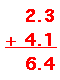Each worksheet has decimal numbers to add.Adding Decimals Path-o-Math Puzzles: 2 by 3Make a path through each number matrix so that the sum of the decimals is equal to the given answer. Use only horizontal and vertical trails. You may not have to use all the numbers, and don't use a number more than once. Puzzle #1, or the answers Puzzle #2, or the answers Puzzle #3, or the answers Puzzle #4, or the answers Puzzle #5, or the answers Subtracting Decimals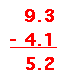Each worksheet has decimal numbers to subtract. Multiplying Decimals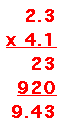Each worksheet has decimal numbers to multiply. Dividing Decimals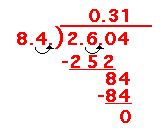Each worksheet has decimal numbers to divide. Express Fractions as Decimals and as Percents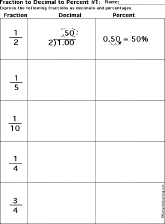Each worksheet has fractions to express as decimals and percentages. Express Decimals as Fractions and as Percents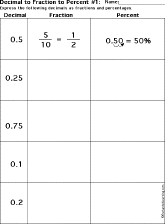Each worksheet has decimals to express as fractions and percentages. Express Percents as Fractions and DecimalsEach worksheet has percentages to convert to decimals and fractions.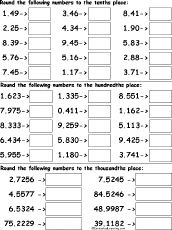Round Decimal Numbers to the Nearest Tenth, Hundredth, or Thousandth DigitRound off the numbers to the nearest tenth digit, hundredth digit, or thousandth digit. Worksheet #1 or go to the answersWorksheet #2 or go to the answersWorksheet #3 or go to the answers.Round Decimal Numbers to the Nearest Tenths, Hundredths, and Thousandths Digit in a GridOn a grid, round off the decimal numbers to the nearest tenths digit, hundredths digit, and thousandths digit. Worksheet #1 or go to the answers Worksheet #2 or go to the answers Worksheet #3 or go to the answers

 +, - EnchantedLearning.comMath x, ÷
 A B C D E F G H I J K L M N O P Q R S T U V W X Y Z
 Number Line Fractions Decimals Measurement Rounding Graphs

Enchanted Learning®
Over 35,000 Web Pages
Sample Pages for Prospective Subscribers, or click below

 Overview of Site What's New Enchanted Learning Home Monthly Activity Calendar Books to Print Site Index K-3 Crafts K-3 Themes Little ExplorersPicture dictionary PreK/K Activities Rebus Rhymes Stories Writing Cloze Activities Essay Topics Newspaper Writing Activities Parts of Speech Fiction The Test of Time iPhone app TapQuiz Maps - free iPhone Geography Game Biology Animal Printouts Biology Label Printouts Biomes Birds Butterflies Dinosaurs Food Chain Human Anatomy Mammals Plants Rainforests Sharks Whales Physical Sciences: K-12 Astronomy The Earth Geology Hurricanes Landforms Oceans Tsunami Volcano Languages Dutch French German Italian Japanese (Romaji) Portuguese Spanish Swedish Geography/History Explorers Flags Geography Inventors US History Other Topics Art and Artists Calendars College Finder Crafts Graphic Organizers Label Me! Printouts Math Music Word Wheels

## Enchanted Learning Search

 Search the Enchanted Learning website for: